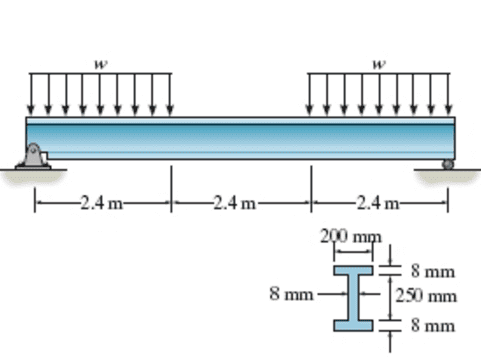# Determine the absolute maximum bending stress of the beam.

• Sarah Henly

## Homework Statement

If w = 74 kN/m , determine the absolute maximum bending stress in the beam in MPa.

## Homework Equations## The Attempt at a Solution

I = [(1/12)*(200*256^3)] - [(1/12)*(192*250^3)] = 29.62*10^6
F = 74*24*1000? [/B]

I assume that F is the upward force in Ns at each end, but it's 2.4m not 24m.
Pick a point at distance x <2.4m from one end. What is the moment of all the forces on one side of it about that point?

## Homework Statement

If w = 74 kN/m , determine the absolute maximum bending stress in the beam in MPa.

## The Attempt at a Solution

I = [(1/12)*(200*256^3)] - [(1/12)*(192*250^3)] = 29.62*10^6
F = 74*24*1000? [/B]
The calculation for I is wrong. This beam has an overall depth of 266 mm, not 256 mm.

The calculation of the equivalent force at each end of the beam is wrong. w = 74 kN / m and extends over a distance of 2.4 m at the ends. You can't ignore those pesky decimal points.

You should get in the habit of recording units with the results of each of your calculations. It eliminates confusion.

The first thing to do is to draw a free body diagram of the beam and find the reactions at each end which keep the beam in equilibrium.

Once you know all of the loadings on the beam, you can construct the shear force and bending moment diagrams to determine the max.bending stress.